# Condition number

Jump to: navigation, search

## Definition for a function of one variable

### For an arbitrary function of one variable

The condition number for a function$f$ at a point$x_0$ in the interior of its domain, and where$f$ is a continuous function, can be defined formally as:$\lim \sup_{x \to x_0} \left| \frac{\frac{f(x) - f(x_0)}{f(x)}}{\frac{x - x_0}{x}} \right|$

where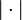$| \cdot |$ denotes the absolute value.

### For a differentiable function of one variable

Consider a function$f$ of one variable. The condition number of$f$ is defined as the absolute value of the relative logarithmic derivative of$f$. Explicitly, the condition number of$f$ at a point$x_0$ in the domain of$f$ satisfying the conditions that the derivative$f'(x_0)$ exists,$x_0 \ne 0$, and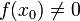$f(x_0) \ne 0$, simplifies to: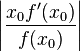$\left|\frac{x_0 f'(x_0)}{f(x_0)}\right|$

In cases where$f'$ is continuous at and around$x_0$, we may be able to compute the limit of this expression to obtain that condition number in cases where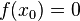$f(x_0) = 0$. Explicitly: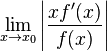$\lim_{x \to x_0} \left|\frac{xf'(x)}{f(x)}\right|$

### For a function with one-sided derivatives

For a function that is not differentiable but has one-sided derivatives$f'_-(x_0)$ and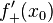$f'_+(x_0)$ at a point$x_0$, the condition number can be defined as: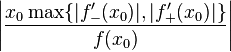$\left|\frac{x_0 \max \{ |f'_-(x_0)|,|f'_+(x_0)| \}}{f(x_0)}\right|$

## Some example functions

Function$f$ (in terms of input variable$x$) derivative$f'$ relative logarithmic derivative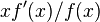$xf'(x)/f(x)$ condition number (itself a function of$x$) limiting value as$x \to \infty$
power function$x^r$ for some real number$r$ (domain conditions apply)$rx^{r-1}$$r$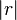$|r|$ (note that the condition number is in this case a constant function)$|r|$
exponential function$e^x$$e^x$$x$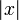$|x|$$\infty$
logarithm function$\ln x$ ($x > 0$)$1/x$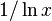$1/ \ln x$$1/|\ln x|$ 0
sine function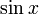$\sin x$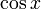$\cos x$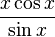$\frac{x \cos x}{\sin x}$$\frac{|x \cos x|}{|\sin x|}$ undefined, fluctuates wildly